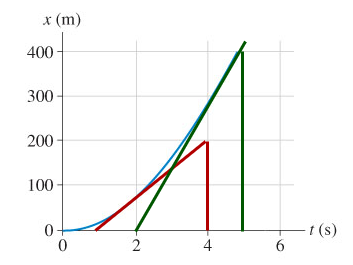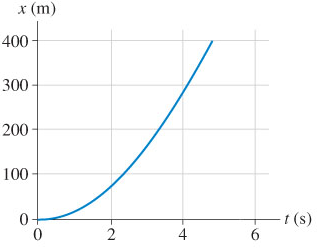# Problem: The figure shows a graph of actual position-versus-time data for a particular type of drag racer known as a "funny car." (Figure 1)Figure 1 of 1Part AEstimate the car's velocity at 2.0 s.Express your answer to one significant figure and include the appropriate units.Part BEstimate the car's velocity at 4.0 s.Express your answer to two significant figures and include the appropriate units.

###### FREE Expert Solution$\overline{){\mathbf{v}}{\mathbf{=}}\frac{\mathbf{∆}\mathbf{x}}{\mathbf{∆}\mathbf{t}}}$

Part A

87% (353 ratings)###### Problem Details

The figure shows a graph of actual position-versus-time data for a particular type of drag racer known as a "funny car." (Figure 1)

Figure 1 of 1Part A

Estimate the car's velocity at 2.0 s.

Express your answer to one significant figure and include the appropriate units.

Part B

Estimate the car's velocity at 4.0 s.

Express your answer to two significant figures and include the appropriate units.